# LEDs and analog drivers for them

2019-04-08

Light-emitting diodes! They're all over our electronic equipment; but what are they all about, really? The underlying concepts are simple, but there are a lot of frequently-asked questions that show there's confusion among hobbyists about how LEDs work and how to apply them in circuits. Here are some notes on that.

### LEDs are diodes

The first thing to understand about LEDs is that they are diodes. If we ignore the "light-emitting" aspect, they function just like any other diodes. They have different values for some of the parameters (just as a big rectifier diode will have different numbers from a 1N4148 switch) but it's the same behaviour.

If you read my article on exponential converters you will know that the current through a forward-biased semiconductor junction is well approximated by an exponential function. Each time you add a certain fixed voltage to the drop across the diode, you multiply the current by a certain amount. In the case of a typical silicon diode, including the forward-bised "diode" formed by the base-emitter junction in an amplifying transistor, the constants are such that adding 18mV doubles the current. For a typical jelly-bean red LED, it takes more like 93mV to double the current; but it's the same kind of curve.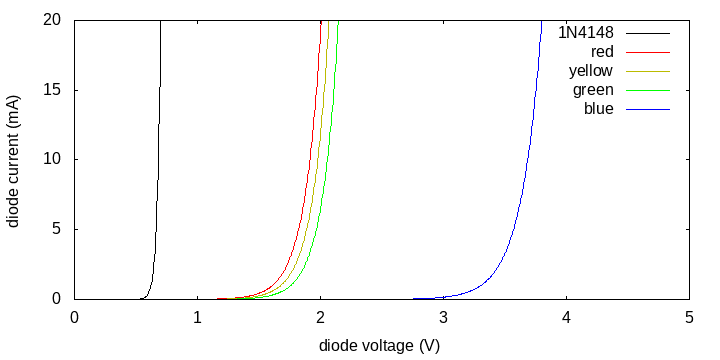Exponential voltage/current behaviour means that for low voltages, the current is basically negligible. Then there is a certain voltage at which all those multiplications finally amount to a significant current. The diode suddenly "turns on," and then if driven beyond that point, it runs away. Small changes in voltage around the turn-on voltage equal large differences in current; and if the voltage goes even a little higher, the current becomes so unreasonably large as to destroy the diode. Flipping the analysis the other way, we can say that for a forward-biased diode the voltage is basically constant. If you know the diode is passing more than negligible current, and you can see that it's not exploding, then you know the forward voltage must be pretty close to where the curve goes vertical, even if you don't know just how much current is flowing. That's why we can talk about "diode drops" and analyse circuits containing 1N4148 silicon diodes on the assumption that they drop about 0.7V. They only really have three states: turned off, turned on and dropping about 0.7V, or destroyed.

The forward voltage depends on the specific diode, the temperature, and some other things, but the biggest determining factor is the material the diode is made out of. All plain silicon diodes drop about 0.7V. All germanium diodes drop somewhat less, like maybe 0.25V. The red, yellow, and green diodes on my chart (which represents typical values but not specific devices) are assumed to be gallium arsenic phosphide combined with gallium phosphide, and have forward voltages of about 1.9V (but, as you can see, there's still some variation). The blue diode is gallium nitride combined with silicon carbide and has a forward voltage of about 3.5V. If you know the material a diode is made out of, you can guess the forward voltage reasonably accurately just from the material.

But you cannot drive a diode just by connecting it to a fixed voltage. If you try, you run into the same problem that happens with synthesizer exponential converters. The shapes of these curves are mostly determined by the material of the diode, but also significantly determined by temperature. Even a single individual diode will have an unpredictable current/voltage curve because you cannot know how the temperature will change. At one moment your LED might be running fine with a voltage drop of 1.9V, then a few moments later it warms up and the preferred voltage drop becomes 1.8V. Now your fixed 1.9V supply intercepts the diode's curve much higher up, the current more than doubles, and the diode may be destroyed. If it survives that, maybe later it cools down a few degrees; the preferred voltage is now 2.0V, and your 1.9V supply doesn't turn the LED on at all. We need a driver circuit that can adjust to different voltages, and I'll discuss that below.

### LEDs by colour

The different diodes on my forward-voltage chart are arranged in rainbow order: red has the lowest voltage, then yellow, then green, and finally blue has the highest voltage. It's no coincidence: the mechanism by which LEDs emit light is intimately connected to voltage. Some fraction of the electrons passing through the device are at high energy levels on one side of the junction, drop to lower energy levels as they cross, and each time that happens, the energy the electron loses is turned into a photon of light.

The electrons can't just drop from any amount of energy to any lesser amount, creating photons spread out over an arbitrary range of energies. Quantum mechanics dictates that electrons in this kind of situation can only carry amounts of energy from a specific list of permitted energy levels. In a semiconductor junction, the possible levels of energy for an electron are arranged into two tight "bands" with a gap of forbidden energy levels in between - the famous "band gap." The light emitted by an LED comes from electrons dropping across the band gap (as they recombine with "holes"); and that same band gap is connected to the forward voltage of the junction. So an LED constructed to have a higher forward voltage will also have a wider band gap and will emit light with more energy per photon; these three things go together. And "more energy per photon" just means "further toward the blue end of the spectrum."

You may note that blue light is not really very much different from green light, so why is the curve for the blue LED so far away from that for the green LED, in comparison to the differences among red, yellow, and green? The answer is that there are other things going on too. The blue LED is made from completely different materials, which have important effects on the forward voltage. But it remains that the materials needed to make shorter-wavelength (bluer) light will tend to also be those that cause the forward voltage to be higher. Colour is strongly connected to voltage even though it is not the only determining factor of voltage.

You may also note that there are other colours of light beyond those we can see. If we had a diode with a forward voltage of less than 1.7V, might it produce light that is beyond red in the red direction, that is, infrared light? And since we do have exactly such a diode plotted on my chart, namely the humble 1N4148, shouldn't it be producing infrared light? In fact, it is. Silicon switching diodes like the 1N4148 are not optimized for maximum light output like LEDs, but they do produce significant amounts of longwave infrared light, which is normally absorbed by their glass or plastic packaging. If you could take one out of its package and image it with a camera capable of detecting far infrared, you'd see it light up like any other LED. LEDs are really just diodes and vice versa; the ones we don't call "light-emitting" are just the ones that are not very efficient at emitting light, are doing it at wavelengths we can't see, or both.

### Reverse voltage

One of the defining things about other diodes is that they only conduct in one direction. The curves above are for forward bias. What happens if you apply voltage to an LED in the opposite direction?

Pretty much exactly the same thing that happens if you apply voltage to any other diode in the opposite direction. For low reverse voltages, no current flows except maybe a small reverse leakage current. If the reverse voltage gets high enough, the LED undergoes breakdown, and will pass a large current probably for a very short time, with permanent damage and smoke emission. They're not like Zeners, capable of running in breakdown mode for extended periods without damage.

As discussed with reference to transistors in my guide for the perplexed, the reverse voltage of a standard silicon junction is related to doping. More heavily doped junctions (which you sometimes need to have for other reasons) have lower breakdown voltages. With LEDs it's more complicated because LEDs are often heterojunction devices, with not just different doping but actually different compound semiconductor materials on the two sides of the junction. But in practice that construction makes them behave as if they were heavily doped. They have low breakdown voltages compared to switching diodes. For a traditional LED with about 2V forward voltage, the maximum reverse voltage may be only about 5V. The newer, bluer, LEDs may have reverse voltage ratings just barely greater than their forward voltages, and they are best treated as "static-sensitive" because even a small amount of charge (which leaks away quickly) beyond the breakdown voltage can damage them permanently.

You can protect an LED against reverse breakdown by putting another diode across it in the opposite direction. When the LED is reverse biased, the protective diode will be forward biased and (as long as the current is low enough not to blow out the protective diode) it will limit the reverse voltage seen by the LED. You can even use two LEDs to protect each other this way; and if they are different colours, you get the traditional kind of tri-state LED, as seen on the North Coast Fixed Sine Bank.  Each "LED" is actually two, in a single jelly bean package with just two legs. Apply current in one direction and the red one glows. Apply current in the opposite direction and the green one glows. And if you switch between the two fast enough, persistence of vision means humans will perceive it as red and green combined, which makes the LED look yellow. The tri-state LED is the first step to the multiplexing of complicated microprocessor-driven LED displays.

If an LED is reverse biased, but not at its breakdown point yet, something interesting happens: the tiny reverse leakage current it supports will be light-sensitive. Every light-emitting diode is also a light-detecting diode! Forrest M. Mims III, whom those of us of a certain age will remember as the author of a series of books on electronics tinkering published by Radio Shack, is generally credited with discovering this effect. Getting the parameters right requires some experimentation with the specific LEDs you're using, but if you want to build a light-sensitive circuit, especially if you want to do it without using RoHS-banned cadmium sulfide photoresistors, you can just put a nondestructive reverse bias across an LED and measure the leakage current.

### Driving an LED with a resistor

You can't just connect an LED to a fixed voltage source, because you can't get the voltage right. With current increasing exponentially as voltage increases, even a tiny error in voltage means the current will be much more or much less than you want. And even if you get the voltage right for one moment with extremely precise and stable control, the LED's current/voltage curve will change from moment to moment (for reasons of temperature and a few other things) and then your carefully chosen fixed voltage will be wrong. So a fixed-voltage driver doesn't work; and that's a problem because in electronic design we usually deal with fixed-voltage supplies, and we'd really like to be able to use one to power an LED.

One way to understand the problem is that, at least near its preferred forward voltage, the LED looks like a fixed voltage source itself, with a very low series resistance. Connect that to another fixed voltage source with a low series resistance, and if the voltages are not exactly the same, you get a lot of current flowing. So if the problem is too little resistance, why not add some in series? That gives one of the simplest safe ways of lighting up an LED, and this circuit is common in practice. The voltages and resistance will of course depend on the application, but these are typical.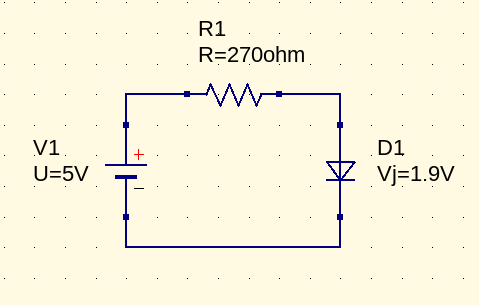If we assume everything is exactly as shown, the power supply offers 5V. The LED drops 1.9V. That leaves 3.1V across the resistor. By Ohm's Law, the current through the resistor is 11.5mA.

But suppose the LED's voltage drop changes to 1.8V. Then there is 3.2V across the resistor and the current increases to 11.9mA.  If the LED's voltage drop moves in the opposite direction, to 2.0V, then we have 3.0V across the resistor, and the current will be 11.1mA.  The current doesn't actually change very much at all. Unlike the LED alone, which can go from almost no current to so much that it explodes in the space of just a small fraction of a volt, this circuit with the resistor keeps a nearly constant current in the face of reasonable changes in the LED parameters.

We can get some idea of what's going on by adding a line to the chart to show the behaviour of the resistor. The voltage across the resistor is always 5V minus whatever is across the LED. So on this chart, where the horizontal axis is the LED voltage, the resistor voltage is 5V at the left edge, decreasing to 0V at the point where the LED voltage reaches 5V. The current through the resistor is directly proportional to the voltage across the resistor, so it decreases in a straight line.   And then the circuit will necessarily operate at a point where the resistor's line intersects the LED's curve.  By looking for the intersections we can accurately predict the current through the diode for any diode we might substitute into the circuit. It's very much like a chart of supply and demand in economics.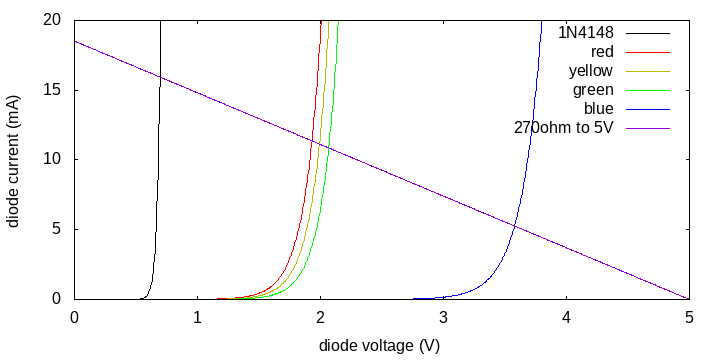If the LED's properties change a little, that has the effect of shifting its curve a little to the left or right. We can see that that won't move the intersection point much, so the current will remain nearly constant, provided that the resistor line is nearly horizontal. If we were to drive this from a lower-voltage supply, we would need a smaller-valued resistor, and then the line for the resistor would slope more. Changes in the LED curve would then have more effect; the whole thing would be less well-controlled. Similarly, if we want to drive a blue LED with 10mA, we're going to need a smaller-valued resistor, and then the line will slope more to hit 5V, and the stability of the current control will be worse.

The basic resistor circuit for lighting up an LED works best if the resistor can drop as much voltage as possible compared to the LED; that implies a higher supply voltage, higher resistance, and more power consumed in the resistor. Driving it from a +12V supply will give more stable control of the current than driving it from a +5V supply. That has to be balanced against what supplies are available, and the power consumption, both from the point of view of saving energy and because if the resistor dissipates a lot of power, it may need to be a physically bigger resistor than is convenient.

Here's a design procedure for the basic resistor driver:

1. Figure out the operating point for the LED: desired current and expected forward voltage at that current. Figure out the power supply voltage.
2. Subtract the LED voltage from the power supply voltage. The result is the voltage across the resistor. Divide this by the desired current to find the target for series resistance. Choose a convenient preferred value near the calculated one.
3. Reverse the calculation: divide the resistor voltage by the chosen resistor value to get the current. Make sure it is acceptable for the LED. Multiply resistor current by resistor voltage to get the power dissipation in the resistor; you must use a resistor rated for at least that much power.

For example, suppose I want to drive a blue LED with a forward voltage of 3.5V at 10mA from a 12V supply. (I'll assume the LED is capable of running at much more, like at least 20mA; we usually don't run them near their maximum current among other reasons because they're often annoyingly bright under such conditions.) The voltage across the resistor is (12.0V-3.5V) = 8.5V. Dividing that by 10mA gives 850 ohms. Let's try 820 ohms as a nearby preferred value. Dividing 8.5V by that gives 10.4mA, which I decide is close enough. The power dissipation will be 10.4mA times 8.5V, which equals 88.1mW, so even a little 1/8W resistor (125mW) will be fine. If these numbers were closer to the limits of the components, I might do a more cautious calculation of the maximum and minimum tolerance values for each of the numbers to make sure there'd be no way the circuit might operate outside of specification.

You can get pretty far with just the basic resistor driver for an LED. In the Transistor ADSR I even used one with a variable input voltage to imitate what an op amp current driver does, without using an op amp. To make it work without placing too much load on the circuit, I had to choose a high-efficiency LED that would be bright enough on just a fraction of a milliamp; it helped that in this application I didn't actually want it to be very bright, not even at maximum, so the LED can run at just a fraction of its specified current rating.

### Analog current drivers

What we're really getting at by adding a resistor to the voltage source, is that we're making the constant-voltage source approximate the behaviour of a constant-current source. The voltage source by itself defines a vertical line on the current/voltage chart. If we had a supply capable of just producing 10mA into whatever load, it would define a horizontal line on the chart, which is the ideal we approach by adding resistance and hoping for the resistance and the input voltage to be high. But with active electronics, we can do better than just adding a resistor. We can get something that comes close to an ideal fixed current output that adjusts to variable load voltage. Here are a few ways to get a more stable constant or near-constant current through the LED.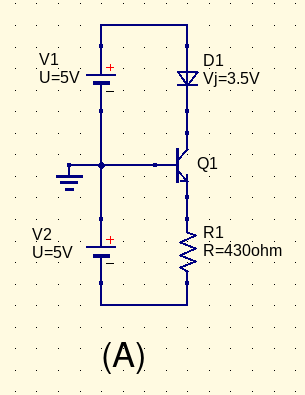In schematic (A), we've got a transistor in a common-base configuration. The resistor R1 is across the negative power supply (5V) minus the base-emitter drop of Q1 (about 0.7V) for a total of 4.3V. That sets the current through the resistor and thus through the emitter of Q1 at 10mA. The base current is a small, and more importantly, a more or less constant, fraction of the emitter current; all the rest must flow through the collector and the LED, D1. What's the advantage compared to just using R1 as a series resistor for the LED? In this circuit, it is a series resistor setting a more or less constant current through the 0.7V base-emitter drop of Q1 instead of through the much larger 3.5V forward voltage of the LED. So there's more stability here. The base-emitter drop cannot change as much as the LED drop could, and the resistor's drop is larger in comparison to it; both factors mean that the current will be less variable. As disadvantages, this circuit is more complex, and it requires a dual power supply. But it's a start.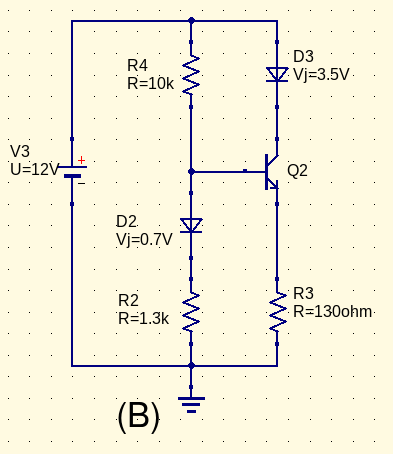Schematic (B) shows a fairly standard single-transistor current source design. The base of Q2 is held at about 2.0V, made up of one standard silicon diode drop of 0.7V plus 1.3V corresponding to 1mA of current through R2. The emitter of Q2 will then float to 1.3V above ground also, which puts 10mA of current through R3, and therefore roughly 10mA through the LED D3 as well. There are some inaccuracies as a result of the base current, possible differences in temperature and certain differences in current between D2 and the base-emitter junction of the transistor, and so on. But this circuit will compensate for most of the temperature variation, at least, and the other factors are more or less constant proportions, so with a little tweaking, it's good at keeping a constant current through the LED.

A hidden benefit of circuit (B) is that it may be able to drive several LEDs in series, as long as their forward voltages don't add up to too many volts for the power rails. It could only support two 3.5V LEDs with the power supply shown, but with redder LEDs that had a smaller forward voltage, or if placed between the +12V and -12V lines of a Eurorack power supply, it could do more. And the efficiency in such a case could be a fair bit better than any of the previously-shown drivers, because the bias components (R2, R4, D2) run at only a fraction of the LED current, while the transistor and R3 run at full current but only a fraction of the voltage used by the LEDs. The simple resistor, or the common-base circuit derived from it, both need to keep the efficiency low in order to keep the voltage drop across the current-setting resistor high.

We can eliminate some of the issues of single transistors by using a whole operational amplifier as a current driver. Here's a simple example.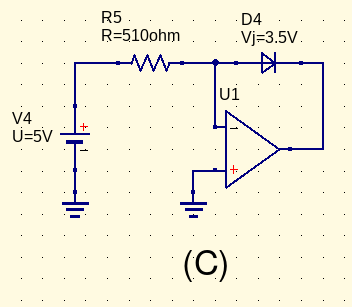In (C), the current is set to 9.8mA (approximately 10mA, but I wanted to use a standard resistor value) by R5 to virtual ground at the negative input of the op amp. Unlike the previous two circuits, there is no need to worry about the size of a diode drop; this resistor will have exactly 5V across it to within the offset specification of the op amp. Then by the basic rules of op amp analysis, no current flows through the op amp input, so the same 9.8mA must flow through D4 as well, regardless of the voltage D4 needs, moment by moment, to pass that current.

If we are just turning on an LED, we don't need the precision of circuit (C). The big advantage of this circuit is that it's voltage-controlled. V4 might not really be a fixed 5V power supply as shown. It could be any source of voltage, and it could be variable. The current through the LED will exactly track whatever voltage V4 assumes; and since light output is basically directly proportional to current, we get the LED going bright or dim in exact proportion to the input voltage. It would not work to just apply the voltage directly to the LED; as discussed, the exponential response of the LED would make it go from basically turned off, to destroyed, over a small and unpredictable range. This circuit gives well-behaved linear control.

One thing to watch out for is that if the input voltage to this circuit were to go negative, then the op amp would cheerfully swing to its maximum positive output voltage, applying that much across the LED in an effort to drive current backward through it. That would probably destroy the LED. If there is any possibility of this circuit receiving negative input, then the LED needs to be protected with a regular diode - or even with a second LED, which then becomes an indicator of negative polarity. It's also necessary to think about the maximum positive input voltage, make sure that that won't create too much current through the LED, and so on.

A potentially serious disadvantage of circuit (C) is that all the current of the LED must flow through R5 and be supplied by the voltage source shown as V4. If that is a fixed voltage it may be no big deal, but as I just got through saying, this circuit is one we might want to use with a variable input voltage to make a variable-brightness LED driver. And many interesting voltage sources (for instance, typical Eurorack signals) will not comfortably drive the 510-ohm input impedance of this circuit. Swapping out the LED for a high-efficiency type that may be able to get as bright as we want on less than 1mA can help; but given we are already involving an op amp, can't we make use of the op amp's amplification capability somehow?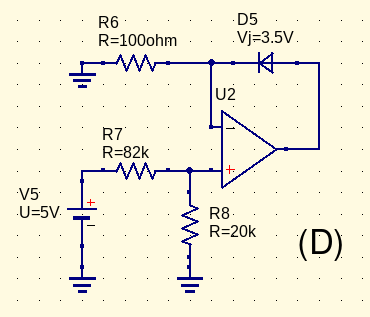In circuit (D), the op amp has been rearranged into a non-inverting configuration (note the reversed direction of the LED). Input voltage goes through a voltage divider to scale it down to about 1V for 5V input. Then the op amp drives the LED as necessary to impose the same voltage across R6; at 1V, that will be 10mA. So the input voltage to LED current characteristic of this circuit will be the same as in circuit (C), but the input impedance is much higher. The input current is only about 0.5% of the LED current. This is what you'd want to use if really driving an LED with variable current from something like a Eurorack signal. As with circuit (C), the LED needs reverse-current protection if the control input can possibly go negative, and it's important to be aware of the maximum positive current the circuit may generate, as driven by the maximum possible input voltage.

One might ask why bother using a voltage divider; why not just drive the positive input of the op amp with the input voltage, and scale R6 as appropriate? Depending on the power supply, the op amp, and the intended range of input voltages, that may work. However, there is a potential issue if we want it to work at relatively high input voltages. The op amp must be able to supply full LED current when its own output is at the LED voltage plus the highest voltage that appears across R6. If we want to allow an input voltage of 5V in Eurorack, with 3.5V across the LED, then the op amp has to source a significant amount of current at 8.5V while running from a ±12V supply, and that may be a strain on its capabilities. It's easy to imagine use cases where this issue would be even more significant (lower power supply voltage, higher input, multiple LEDs in series, etc.). Gearing down the input with a voltage divider allows us to keep the drop across R6 and therefore the output voltage of the op amp much smaller. It also saves some power dissipation in R6.

I've described the basic behaviour of LEDs with respect to voltage and current, and some circuits for driving them properly in an analog context - either just turning them on, or making the brightness vary with a control voltage. There is also a lot to be said about driving LEDs in a digital context. In particular, displays made up of multiple LEDs that turn on and off raise some interesting issues both for interfacing to digital circuits, and for managing their effect on the power supply. In some future posting I'll discuss digital LED drive circuits.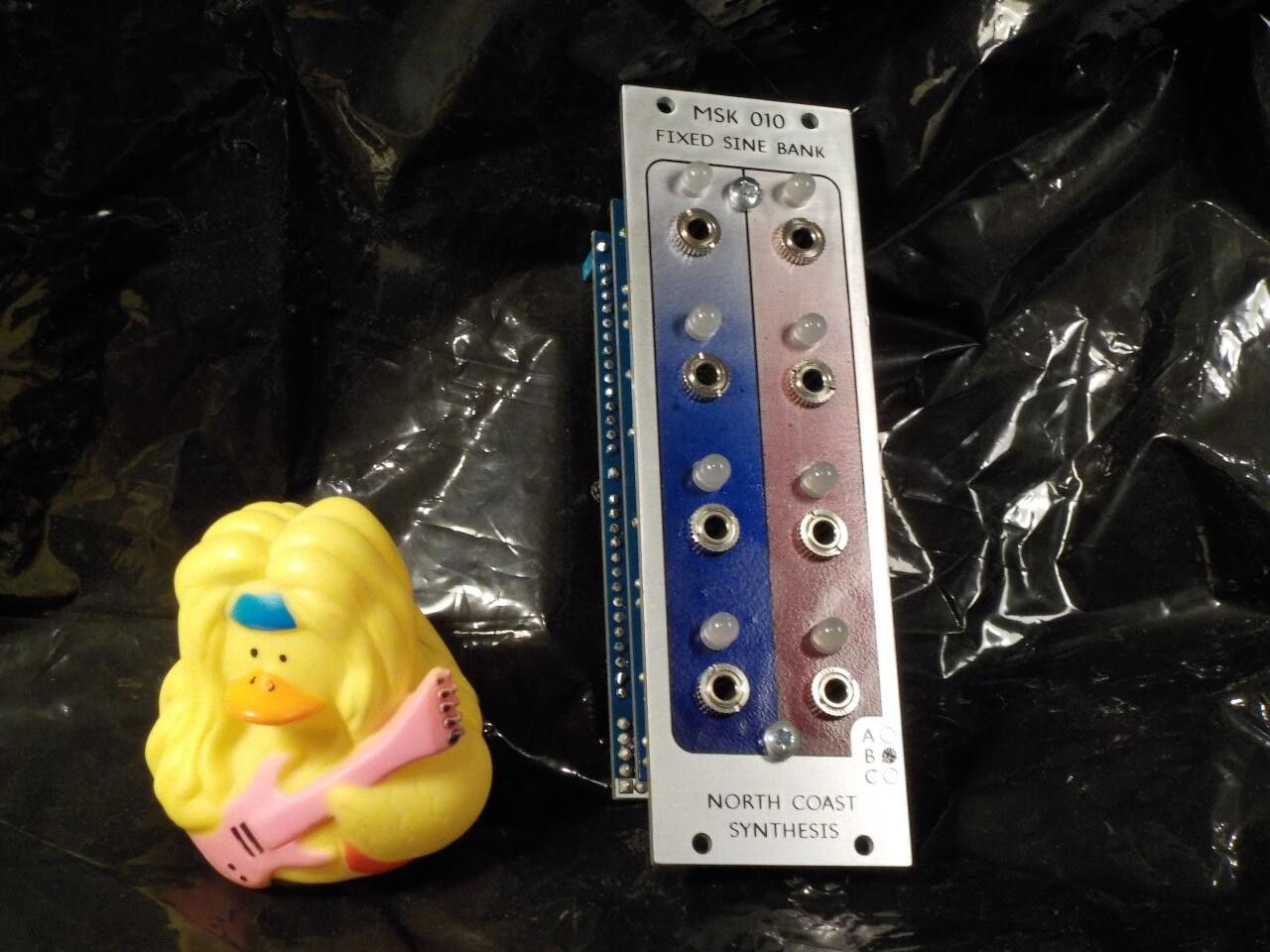## MSK 010 Fixed Sine Bank, variant B

### US\$202.68 including shipping

Anti-spam, fill in the blank: Coast Synthesis